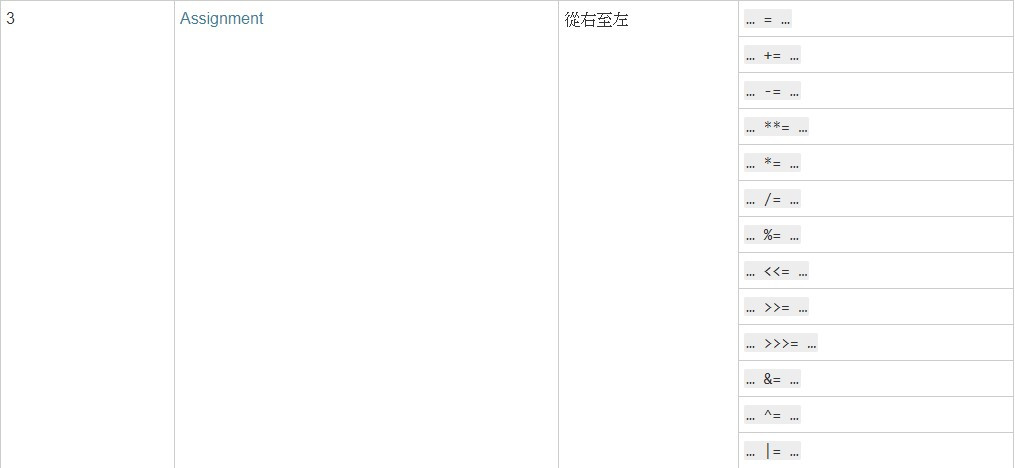#1

## Javascript 進階 3-6 優先性及相依性### 優先性

``````var a = 2 * 2 + 2 * 3;
console.log(a);
``````### 相依性

``````// 先乘除 相依性左到右
2 * 2 = 4
2 * 3 = 6

// 加減 相依性左到右
4 + 6 = 10

// 賦值 相依性右到左
a = 10
``````

``````console.log(1 < 2 < 3); // true
``````

``````console.log(3 > 2 > 1); // false
``````

``````// 小於大於的符號，優先度一樣是11，那再來就是先相依性的順序都為 從左至右，但這裡必須拆開來看
3 > 2 // 回傳 true， true 在javascript 中會自己型別轉換為 1， 相反 false 就是轉換成 0。
// 所以就變成
1 > 1 // 這邊當然是回傳 false
``````

``````var a = 1;
var b = 2;
a = b = 3;
console.log(a, b); // 3 , 3
``````

``````// 因為 = 是由右邊到左邊的相依性，所以會是這樣
b = 3;
a = b;
``````

# 但是，雖然結果是對的，觀念理解卻是錯的喔!!!!!

``````var b = {};
Object.defineProperty(b, 'a', {
value: 2,
writable: false
});
``````

``````var b = {};
Object.defineProperty(b, 'a', {
value: 2,
writable: false
});

b.a = 3; // 2
console.log('b.a', b.a);

var a = 3;
a = b.a = 1;
``````

``````b.a = 1;

// 這裡雖然b.a的值還是2，但這個表達式會 回傳的值 其實是1喔
// 而重點是回傳的值會再次成為其他運算子的運算元，所以接下來的表達式是等同於

a = 1(b.a = 1的回傳值)

// 所以 a 才等於 1喔!!
``````

``````var b = {};
Object.defineProperty(b, 'a', {
value: 2,
writable: false
});

Object.defineProperty(b, 'b', {
value: 3,
writable: false
});

b.a = 3; // 2
console.log('b.a', b.a);

var a = 3;
a = b.b =b.a = 1;
``````

``````var a = 1;
var b = 2;
a = b = 3;
console.log(a, b); // 3 , 3
``````

``````b = 3; // 回傳 3
a = 3(b = 3的回傳值);
``````

### 1 則留言

0
``````console.log(1 > 2 > 3);
``````

``````console.log(3 > 2 > 1);
``````
odinhusky iT邦新手 5 級 ‧ 2019-12-17 08:55:50 檢舉